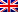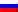# Hopfield Network

Contenus

## Hopfield Network

In a Hopfield lattice, during the formation process, one can think that the weights in the lattice minimize an energy function and slide on an energy surface. In a trained network, each model presented to the network provides an attractor, where progress is made towards the point of attraction by propagating information around the network.

The goal of system information processing is to associate the components of an input model with a holistic representation of the model called Content Addressable Memory (CAM). This means that once trained, the system will recall entire patterns or a noisy version of the input pattern.

The Hopfield network includes a graphical data structure with weighted edges and separate procedures for forming and applying the structure. The network structure is fully connected (one node connects to all other nodes except itself) and the edges (weights) between nodes are bidirectional.

The weights of the network can be learned via a one-shot method (an iteration on all the models) if all the models to be memorized by the network are known. Alternatively, the weights can be incrementally updated using the Hebb rule where the weights are increased or decreased based on the difference between the actual output and the expected output. The one-time calculation of network weights for a single node occurs as follows:

where w_i, j is the weight between neuron i and j, N is the number of input models, v is the input model and v_ik is the i-th attribute on the k-th input model.

The propagation of information through the network can be asynchronous where a random node is selected on each iteration, or synchronously, where the output is calculated for each node before being applied to the entire network. Information propagation continues until no further changes are made or until a maximum number of iterations have been completed, after which the network output template can be read. The activation for a single node is calculated as follows:

where n_i is the activation of the i-th neuron, w_i, j with the weight between nodes i and j, and n_j is the output of the j-th neuron. Activation is transferred to an output using a transfer function, typically a step function as follows:

where the threshold θ is generally set at 0.

The Hopfield grating can be used to solve the problem of recalling cue matches for an entry pattern to an associated pre-learned pattern.

The transfer function to transform the activation of an output neuron is typically a step function f (a) in {-1, 1} (preferred), or more traditionally f (a) in {0, 1}. Input vectors are usually normalized to Boolean values x in [-1; 1].

The network can be propagated asynchronously (where a random node is selected and generated as output), or synchronously (where the output of all nodes is calculated before being applied).

Weights can be learned in a one-time or incremental method depending on the amount of information known about the models to be learned. All neurons in the network are generally both input and output neurons, although other network topologies have been investigated (such as the designation of input and output neurons). A Hopfield network has limits on the models it can store and precisely retrieve from memory, described by N <0.15 * n where N is the number of models that can be stored and retrieved and n is the number of nodes in the network.FRFRENRUES